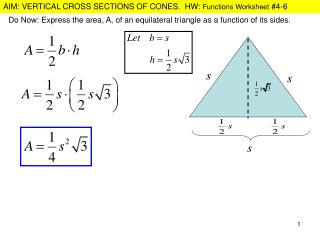# Do Now: Express the area, A, of an equilateral triangle as a function of its sides. - PowerPoint PPT PresentationDownload PresentationDo Now: Express the area, A, of an equilateral triangle as a function of its sides.

Do Now: Express the area, A, of an equilateral triangle as a function of its sides.
Download Presentation## Do Now: Express the area, A, of an equilateral triangle as a function of its sides.

- - - - - - - - - - - - - - - - - - - - - - - - - - - E N D - - - - - - - - - - - - - - - - - - - - - - - - - - -
##### Presentation Transcript

1. Do Now: Express the area, A, of an equilateral triangle as a function of its sides.

2. What is the relationship between the radius of the base and the height of a cone? Let’s observe some things that are true about this diagram: Congruent Triangles: height Similar Triangles: As a result, we may set up the following proportion: Ex) A water tank has the shape of a right circular cone with its vertex pointing down. The radius, r, of the top is 2 ft and the height, h, of the tank is 8 ft. Express the volume of the water in the tank as a function of its depth.

3. PRIMARY SECONDARY Write the radius in terms of h and substitute that radius into the volume equation to get the volume equation in terms of h as well. Ex) A water tank has the shape of a right circular cone with its vertex pointing down. The radius, r, of the top is 2 ft and the height, h, of the tank is 8 ft. Express the volume of the water in the tank as a function of its depth (height).

4. Functions Worksheet #4-6 4. A water tank has the shape of a right circular cone with its vertex pointing downward. The radius of the top is 3 ft., and the height of the tank is 12 ft. Express the volume of the water in the tank as a function of its depth.

5. 5. The vertical cross sections of a conical water tank are isosceles right triangles. Express the volume of the water in the tank as a function of its depth. Vertical Cross Sections: A vertical slicing of a cone creating 2 isos. right triangles. The vertical cross sections of a cone are the triangles that are formed when you slice a cone vertical starting from the tip of the vertex; when you open the cone and look at it head-on you see, in this case, 2 Isosceles right triangles. PRIMARY SECONDARY

6. 6. The vertical cross sections of a conical water tank are equilateral triangles. Express the volume of the water in the tank as a function of its depth. Vertical Cross Sections: A vertical slicing of a cone creating 2 equilateral triangles. SECONDARY PRIMARY

7. FINISH WORKSHEET: #7, 8, 9

8. REIVEW OF FUNCTION APPS: A rectangle is bounded by the x-axis and the semi-circle A Write the area of the rectangle as a function of x and determine the domain of the function. What if the question said that the rectangle is inscribed in the entire circle?